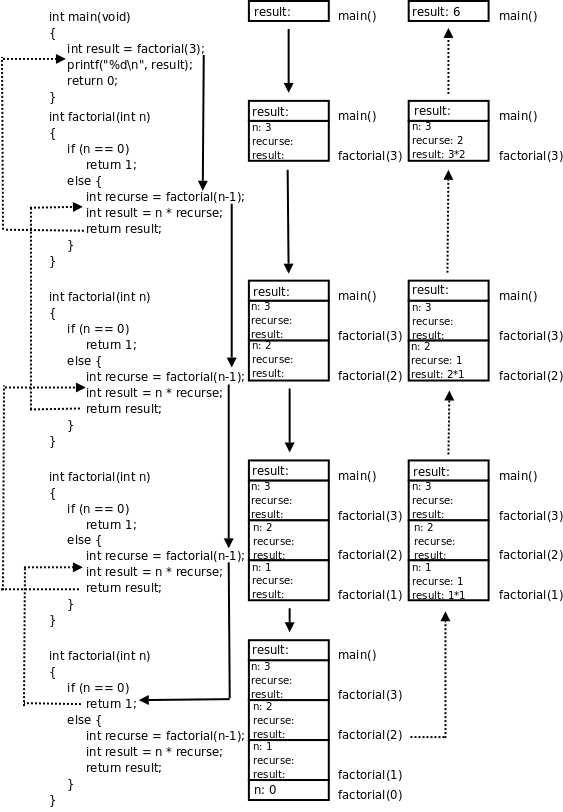## 3. 递归

frabjuous

an adjective used to describe something that is frabjuous.

0! = 1
n! = n · (n-1)!

```int factorial(int n)
{
if (n == 0)
return 1;
}```

```int factorial(int n)
{
if (n == 0)
return 1;
else {
int recurse = factorial(n-1);
int result = n * recurse;
return result;
}
}```

`factorial`这个函数居然可以自己调用自己？是的。自己直接或间接调用自己的函数称为递归函数。这里的`factorial`是直接调用自己，有些时候函数A调用函数B，函数B又调用函数A，也就是函数A间接调用自己，这也是递归函数。如果你觉得迷惑，可以把`factorial(n-1)`这一步看成是在调用另一个函数－－另一个有着相同函数名和相同代码的函数，调用它就是跳到它的代码里执行，然后再返回`factorial(n-1)`这个调用的下一步继续执行。我们以`factorial(3)`为例分析整个调用过程，如下图所示：1. `main()`有一个局部变量`result`，用一个框表示。

2. 调用`factorial(3)`时要分配参数和局部变量的存储空间，于是在`main()`的下面又多了一个框表示`factorial(3)`的参数和局部变量，其中`n`已初始化为3。

3. `factorial(3)`又调用`factorial(2)`，又要分配`factorial(2)`的参数和局部变量，于是在`main()``factorial(3)`下面又多了一个框。第 4 节 “全局变量、局部变量和作用域”讲过，每次调用函数时分配参数和局部变量的存储空间，退出函数时释放它们的存储空间。`factorial(3)``factorial(2)`是两次不同的调用，`factorial(3)`的参数`n``factorial(2)`的参数`n`各有各的存储单元，虽然我们写代码时只写了一次参数`n`，但运行时却是两个不同的参数`n`。并且由于调用`factorial(2)``factorial(3)`还没退出，所以两个函数调用的参数`n`同时存在，所以在原来的基础上多画一个框。

4. 依此类推，请读者对照着图自己分析整个调用过程。读者会发现这个过程和前面我们用数学公式计算3!的过程是一样的，都是先一步步展开然后再一步步收回去。

`return area(distance(x1, y1, x2, y2));`

```...
int recurse = factorial(n-1);
int result = n * recurse;
...```

```int factorial(int n)
{
int recurse = factorial(n-1);
int result = n * recurse;
return result;
}```

### 习题

1、编写递归函数求两个正整数`a``b`的最大公约数（GCD，Greatest Common Divisor），使用Euclid算法：

1. 如果`a`除以`b`能整除，则最大公约数是`b`

2. 否则，最大公约数等于`b``a%b`的最大公约数。

Euclid算法是很容易证明的，请读者自己证明一下为什么这么算就能算出最大公约数。最后，修改你的程序使之适用于所有整数，而不仅仅是正整数。

2、编写递归函数求Fibonacci数列的第`n`项，这个数列是这样定义的：

fib(0)=1
fib(1)=1
fib(n)=fib(n-1)+fib(n-2)

Lamé定理

 例如很多编程书都会举例的汉诺塔问题，本书不打算再重复这个题目了。